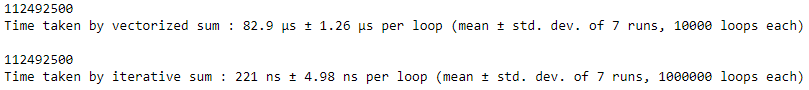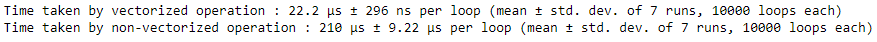# Vectorized Operations in NumPy

Numpy arrays are homogeneous in nature means it is an array that contains data of a single type only. Python’s lists and tuples, which are unrestricted in the type of data they contain. The concept of vectorized operations on NumPy allows the use of more optimal and pre-compiled functions and mathematical operations on NumPy array objects and data sequences. The Output and Operations will speed-up when compared to simple non-vectorized operations.

Example 1 : Using vectorized sum method on NumPy array. We will compare the vectorized sum method along with simple non-vectorized operation i.e the iterative method to calculate the sum of numbers from 0 – 14,999.

 `# importing the modules ` `import` `numpy as np ` `import` `timeit ` ` `  `# vectorized sum ` `print``(np.``sum``(np.arange(``15000``))) ` ` `  `print``(``"Time taken by vectorized sum : "``, end ``=` `"") ` `%``timeit np.``sum``(np.arange(``15000``)) ` ` `  `# itersative sum ` `total ``=` `0` `for` `item ``in` `range``(``0``, ``15000``): ` `    ``total ``+``=` `item ` `a ``=` `total ` `print``(``"\n"` `+` `str``(a)) ` ` `  `print``(``"Time taken by iterative sum : "``, end ``=` `"") ` `%``timeit a `

Output :The above example shows the more optimal nature of vectorized operations of NumPy when compared with non-vectorized operations. This means when computational efficiency is the key factor in a program and we should avoid using these simple operations, rather we should use NumPy vectorized functions.

Example 2 : Here we will compare numpy exponential function with python built-in math library exponential function to calculate the exponential value of each entry in a particular object.

 `# importing the modules ` `import` `numpy as np ` `import` `timeit ` `import` `math ` ` `  `# vectorized operation ` `print``(``"Time taken by vectorized operation : "``, end ``=` `"") ` `%``timeit np.exp(np.arange(``150``)) ` ` `  `# non-vectorized operation ` `print``(``"Time taken by non-vectorized operation : "``, end ``=` `"") ` `%``timeit [math.exp(item) ``for` `item ``in` `range``(``150``)] `

Output :Here as we can see NumPy vectorized operations are more optimized in calculating value and along with one more limitation of Python math library i.e math library range limit, as it not suitable for very large value, unlike NumPy vectorized operation which can be used to calculate the exponential value of very large range limit as well.

The above two examples justify the optimal nature of NumPy vectorized functions and operations when compared and used in place of simple or non-vectorized function or operations in a python program or script.

My Personal Notes arrow_drop_upCheck out this Author's contributed articles.

If you like GeeksforGeeks and would like to contribute, you can also write an article using contribute.geeksforgeeks.org or mail your article to contribute@geeksforgeeks.org. See your article appearing on the GeeksforGeeks main page and help other Geeks.

Please Improve this article if you find anything incorrect by clicking on the "Improve Article" button below.

Article Tags :

Be the First to upvote.

Please write to us at contribute@geeksforgeeks.org to report any issue with the above content.